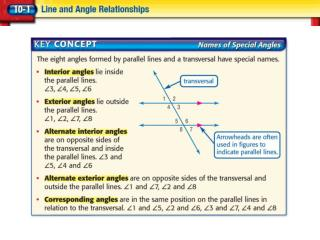DownloadDownload PresentationFind Measures of Angles

# Find Measures of Angles

Télécharger la présentation## Find Measures of Angles

- - - - - - - - - - - - - - - - - - - - - - - - - - - E N D - - - - - - - - - - - - - - - - - - - - - - - - - - -
##### Presentation Transcript

1. Find Measures of Angles In the figure, m|| n and t is a transversal. If m7 = 123°, find m2 and m8. Since 7 and 2 are alternate exterior angles, they are congruent. So, m2 = 123°. Answer:m2 = 123° Since 7 and 8 are corresponding angles, they are congruent. So, m8 = 123°. Answer:m8 = 123°

2. In the figure, m|| n and t is a transversal. If m4 = 57°, find m5 and m1. A.m5 = 57°; m1 = 57° B.m5 = 123°; m1 = 123° C.m5 = 57°; m1 = 123° D.m5 = 123°; m1 = 57°

3. LEG LIFTSKian does leg lifts each morning. For each repetition he lifts his legs 35 degrees off the ground. What is the measure of the angle formed by his body and legs in this position? The angles are supplementary. x + 35 = 180 Write the equation. x = 180 – 35 Subtract 35 from each side. x = 145 Answer: The angle formed by his body and legs is 145°.

4. SEWING Linda cuts a piece of material from the corner at a 35° angle. What is the measure of the other angle formed by the cut? A. 145° B. 90° C. 55° D. 35° 90 - 35

5. Find Measures of Angles ALGEBRAAngles PQR and STU are supplementary. If mPQR = x – 15 and mSTU = x – 65, find the measure of each angle. Step 1 Find the value of x. Step 2 Replace x with 130 to find the measure of each angle. x – 15 + x – 65 = 180° 2x – 80 = 180° 2x = 260° x = 130° x – 15 = 130 – 15 or 115 x – 65 = 130 – 65 or 65 Answer:mPQR = 115° mSTU = 65°

6. Angles ABC and DEF are complementary. If mABC = x + 12 and mDEF = 2x – 9, find the measure of each angle. A.mABC = 29°; mDEF = 61° B.mABC = 41°; mDEF = 49° C.mABC = 59°; mDEF = 121° D.mABC = 71°; mDEF = 109°

7. TRANSPORTATIONA road crosses railroad tracks at an angle as shown. If m1 = 131°, find m6 and m5. Since 1 and 5 are corresponding angles, they are congruent. Answer:m5 = 131° Since 5 and 6 are supplementary angles, the sum of their measures is 180°.180 – 131 = 49 Answer:m6 = 49°

8. TRANSPORTATION Main Street crosses Broadway Boulevard and Maple Avenue at an angle as shown. If m1 = 48°, find m3 and m4. A.m3 = 132°; m4 = 48° B.m3 = 48°; m4 = 132° C.m3 = 48°; m4 = 42° D.m3 = 42°; m4 = 138°